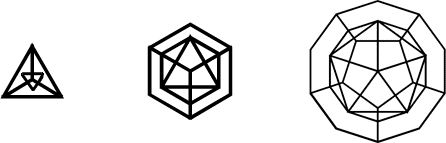## Duality

When the centers of a polyhedrons faces are joined, the new polyhedron that is created is said to be the dual of the original polyhedron.

A quick look on the table of polyhedra will reveal which polyhedra are duals. Whenever the vertices and faces of two polyhedra inversely match, then those two polyhedra are said to be dual. The cube and the octahedron, 8 vertices / 6 faces and 6 vertices / 8 faces, respectively are dual, as is the dodecahedron and icosahedron. The tetrahedron, having equal numbers of vertices and faces, is its own dual.Duality is not a characteristic restricted to only three-dimensional polyhedra. Duality extends to all dimensional analogues of polyhedra. The rules of construction for these analogues work as such: When the centers of a n-dimensional polyhedrons (n-1)-face are joined, the new polyhedron created is said to be the dual of the original. So now, instead of matching vertices to faces, we are matching vertices to (n-1)-faces. For two-dimensional polyhedra, this is vertices to edges, three dimensional polyhedra, vertices to faces, four dimensional polyhedra, vertices to cells, and so on.